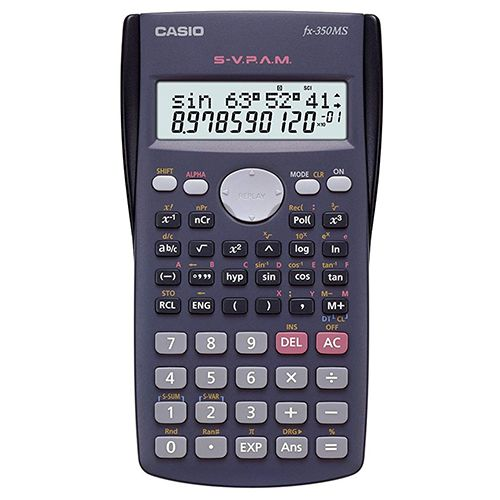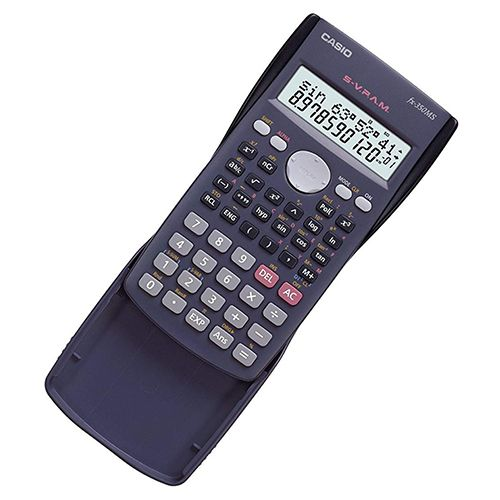USD - \$

# Casio Scientific Calculator fx-350MS## Product Details

### Features:

• Fraction calculations
• Combination and permutation
• Statistics (STAT-data editor, Standard deviation, Regression analysis)
• 9 variables
• Comes with slide-on hard case
• Regular Percent
• Regular percentage calculations
• Multi-Replay
• Quick and easy recall of previously executed formulas for editing and re-execution
• Plastic Keys
• Designed and Engineered for Easy Operation
• S-V.P.A.M. (Super Visually Perfect Algebraic Method)
• All the features of the existing V.P.A.M. series plus a new 2-line display and a useful Replay function. All this helps to make mathematics easier to use and easier to understand than ever before
• STAT-Data Editor
• Back-step viewing and editing of input data
• Basic Mathematical Functions
• Trigonometric, Exponential logarithmic, etc
• Basic Statistics
• Standard statistics functions such as Mean, SUM, Standard Deviation and Regression

### Specification:

• Brand: Casio
• Product Type: Scientific Calculator
• Number of Functions : 240
• Battery: LR44 × 1
• Digit: 10 + 2 digits
• Display Type: Dot Matrix

### Questions?